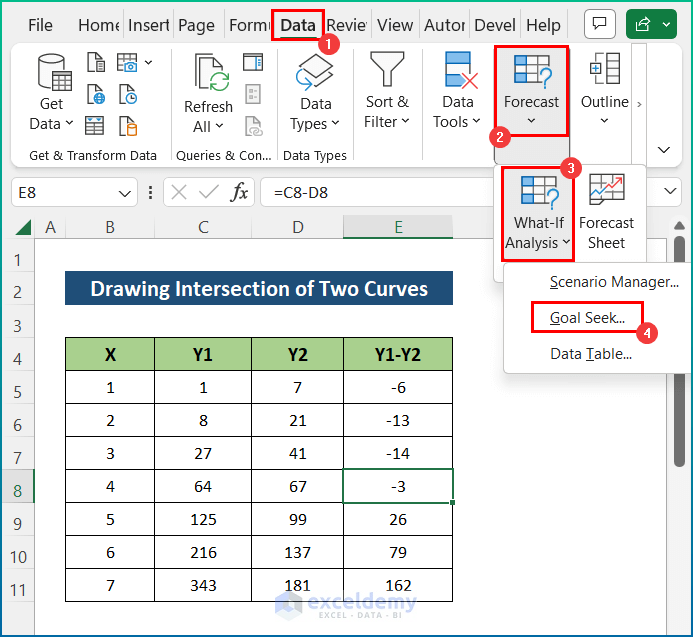# How to Find Intersection of Two Curves in Excel (with Easy Steps)

Get FREE Advanced Excel Exercises with Solutions!

Fortunately, many of us use Excel in our business organizations. In any business organization, we use Excel to organize data as needed and make databases for the future. Moreover, one interesting thing is that we can find the intersection of two curves easily in Excel for better representation. However, I have used Microsoft Office 365 for the purpose of demonstration, and you can use other versions according to your preferences. In this article, I will show you a step-by-step procedure to find the intersection of two curves in Excel. Hence, read through the article to learn more and save time.

## How to Find Intersection of Two Curves in Excel: Step-by-Step Procedures

Often, we need to find the intersection of two curves in certain business analytics, and the process becomes more interesting with Excel. However, the task is easy and simple. But you will need an arrangement in order to perform the operation properly. Hence, go through the following steps to find your desired intersection of two curves using Excel.

### 📌 Step 1: Select Dataset

• For the purpose of demonstration, I have selected the following sample dataset. Here, the dataset contains 3 variables including X, Y1, and Y2.
• Here, we have selected 2 sample equations in order to plot equations in Excel.

`y = 3x``2`` + 5x - 1`

`y = x``3`• Now, select columns X and Y1.
• Then, click the Insert tab. Moreover, click on the Scatter command from the Charts group of commands.
• Next, select the Scatter chart. After some modification, it will look like the image below.• Afterwards, select the graph to Select Data and click on Add.
• Now, provide the X and Y values and press OK.• Finally, the chart which includes the intersection of two curves will appear as below after some modifications.### 📌 Step 2: Calculation to Get Intersection of Two Curves

• Firstly, select cell E5 and insert the following formula to get the difference between Y1 and Y2.

`=C5-D5`• Secondly, hit the Enter key and utilize the AutoFill tool to the entire column.• Finally, you will get all the differences.### 📌 Step 3: Find Intersection Point in Excel

• Initially, select any cell within range E5:E11.
• Then, go to the Data tab and select Forecast.
• Next, select Goal Seek from the What-If Analysis drop-down menu.

###• After that, put 0 in To value. As there is no difference in the Y value of the two curves at the point of intersection.
• Again, select the B8 cell in By changing cell because you have to make the difference 0 by changing the value of X.
• Now, press OK.• Lastly, it will show that it has found a solution and press OK.### Final Output

Last but not least, you will get your desired point of intersection of two curves. However, it will appear on the same row that you have selected in the Goal Seek dialog box. Here, I have found the values of X = 4.15 and Y = 71.34.Here, the difference is less than 10-5 and it tends to be 0. Hence, the solution is correct enough.

However, you can check the value with the graph. From the graph, it is clearly evident that the point of intersection of the two curves is correct.## 💬 Things to Remember

• First of all, there is no direct chart option to get the intersecting point of two curves.
• Then, you can modify the chart according to your personal preference.
• However, you can choose any cell from the difference(Y1-Y2) range and it will provide the same solution every time.
• At last, you can copy a row from the dataset and paste it to a different region in the sheet if you don’t want to overlap the solution with the dataset.

## Conclusion

These are all the steps you can follow to find the intersection of two curves in Excel. Overall, in terms of working with time, we need this for various purposes. I have shown multiple methods with their respective examples, but there can be many other iterations depending on numerous situations. Hopefully, you can now easily create the needed adjustments. I sincerely hope you learned something and enjoyed this guide. Please let us know in the comments section below if you have any queries or recommendations.

## What is ExcelDemy?

ExcelDemy Learn Excel & Excel Solutions Center provides free Excel tutorials, free support , online Excel training and Excel consultancy services for Excel professionals and businesses. Feel free to contact us with your Excel problems.Mehedi Hasan

Hi, I am Mehedi. I have completed my B.Sc. from Bangladesh University of Engineering and Technology. I have a strong interest in innovation and research in the field of Data Science and Machine Learning. Gradually, I now understand the value of Data Analysis and I am trying to learn everyday.

We will be happy to hear your thoughtsAdvanced Excel Exercises with Solutions PDF